Function Repository Resource:

# FindMinimumPlot

Visualize the function evaluations done by FindMinimum

Contributed by: Wolfram Staff (with additional contributions by Jan Mangaldan)
 ResourceFunction["FindMinimumPlot"][f,{x,xst}] plots the steps and the points at which the function f and any of its derivatives are evaluated in FindMinimum[f,{x,xst}], superimposed on a plot of f versus x. ResourceFunction["FindMinimumPlot"][f,{{x,xst},{y,yst}}] plots the steps and the points at which the bivariate function f and any of its derivatives are evaluated, superimposed on a contour plot of f as a function of x and y. ResourceFunction["FindMinimumPlot"][f,range,property] returns the specified property.

## Details

The argument property can be any of the following:
 "Result" the result of FindMinimum "Statistics" an association showing the number of steps and evaluations of the function and its derivatives "Plot" the plot of the function and the evaluation points All association containing "Result", "Statistics" and "Plot"
ResourceFunction["FindMinimumPlot"] takes the same options as FindMinimum and Plot (in the one-dimensional case) or ContourPlot (in the two-dimensional case), with the following addition:
 "EvaluationStyles" Automatic styles used for the evaluation points
The "EvaluationStyles" option takes an association whose values correspond to the styles used for the evaluation points in the plot.
With the setting , ResourceFunction["FindMinimumPlot"] shows a legend for the evaluation points.

## Examples

### Basic Examples (2)

Show the steps and function evaluations used in finding a local minimum of the function exp(x)+1/x:

 In:=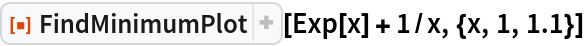Out=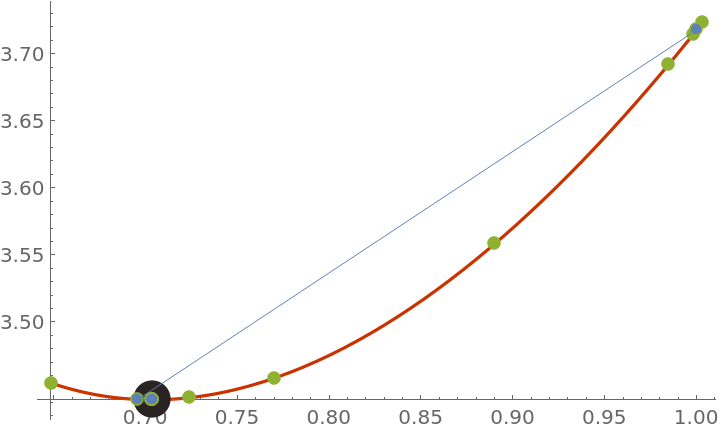Show the steps and function evaluations used in finding a local minimum of the function cos(x2-3y)+sin(x2+y2):

 In:=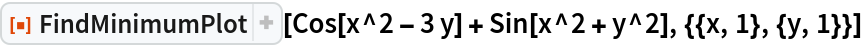Out=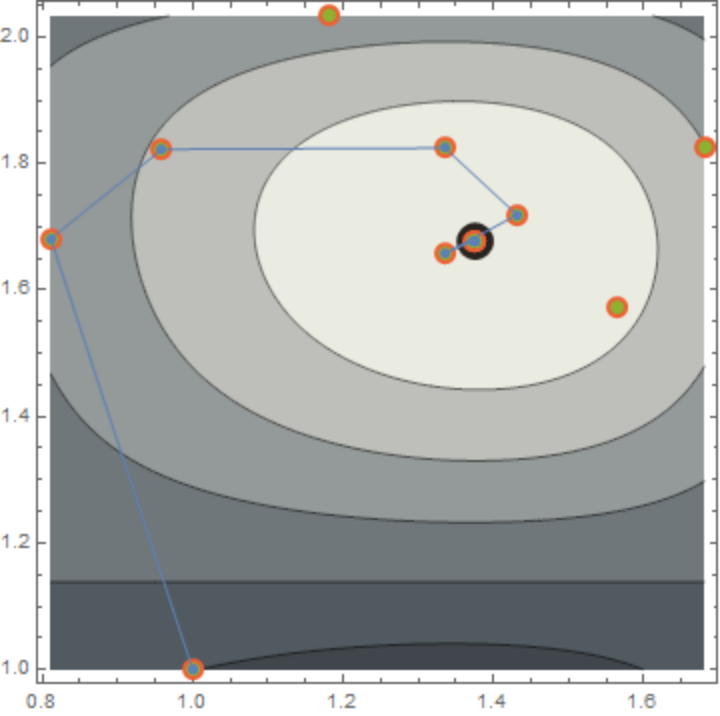### Scope (2)

Use PlotLegends to display a legend for the evaluation points:

 In:=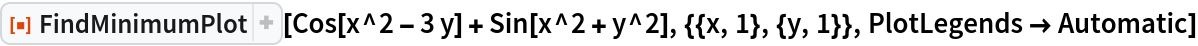Out=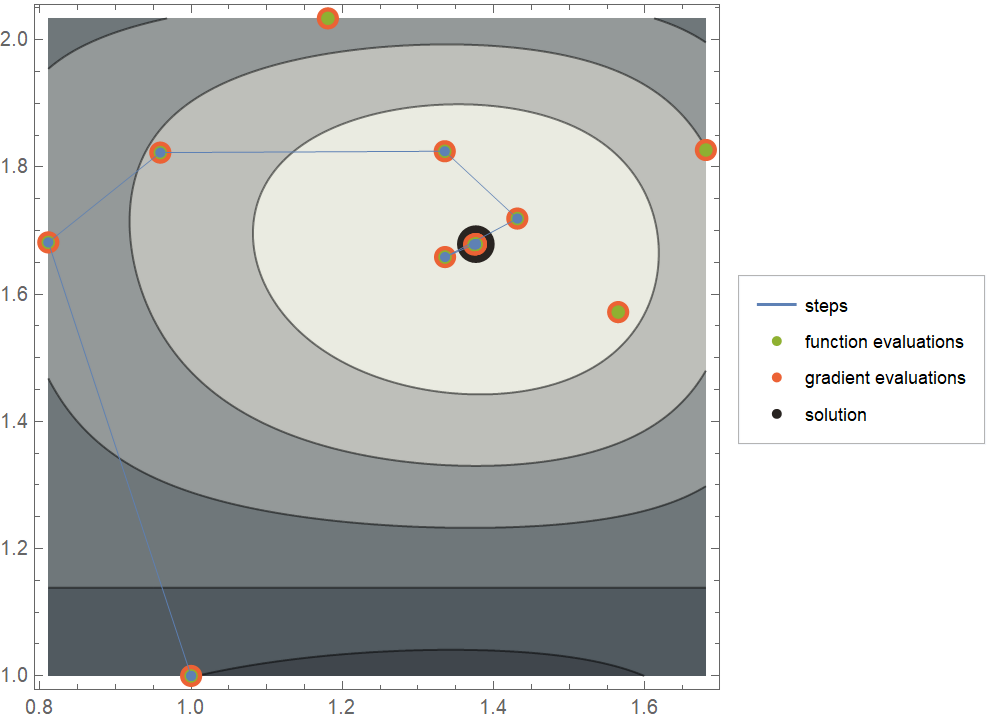Use a different minimization method from the default:

 In:=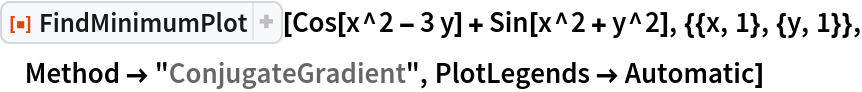Out=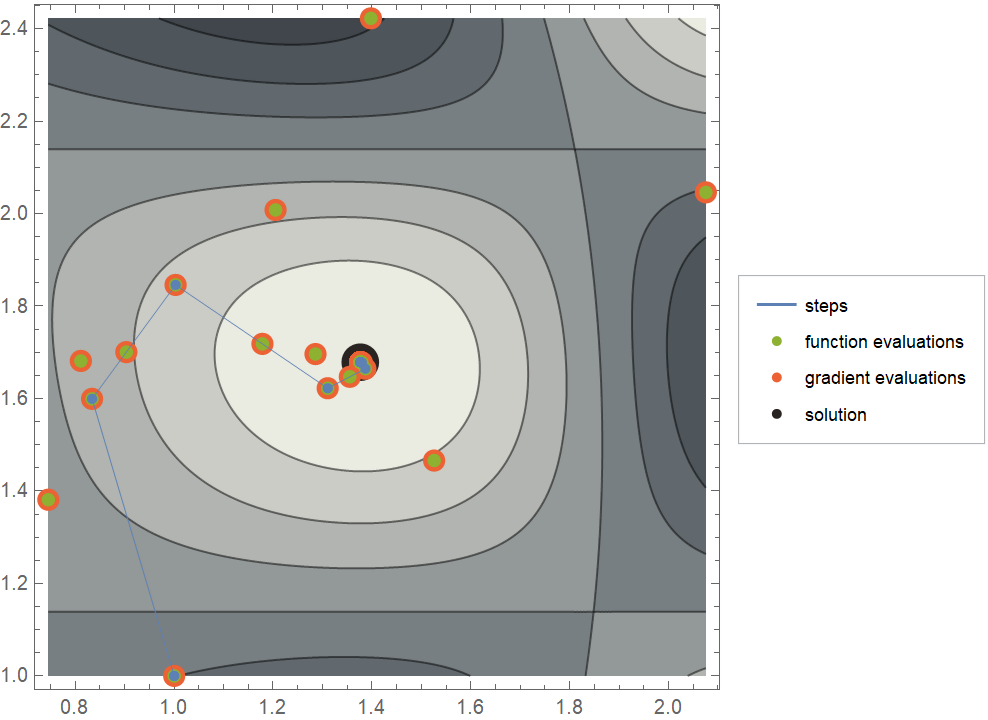Return the statistics of the evaluations done by FindMinimum:

 In:=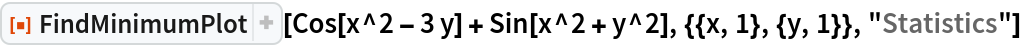Out=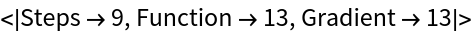Return all properties:

 In:=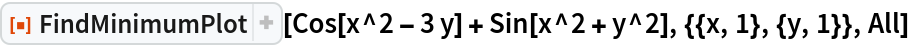Out=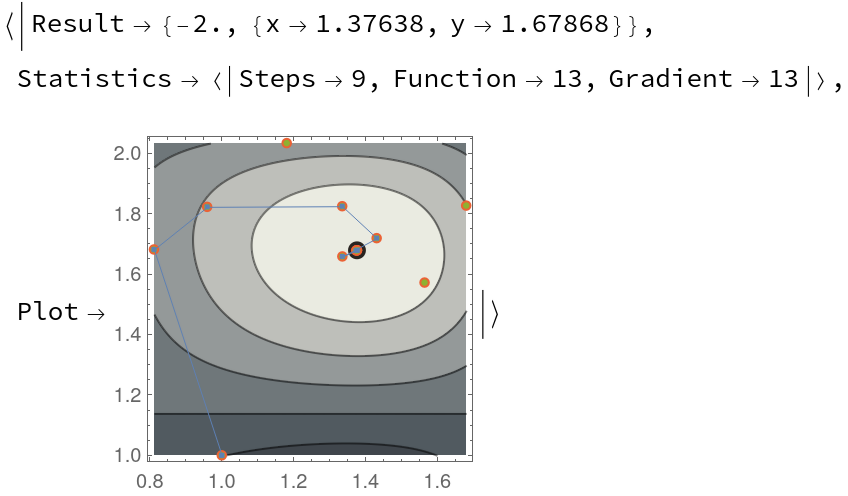### Options (5)

#### ColorFunction (1)

Use a different ColorFunction setting:

 In:=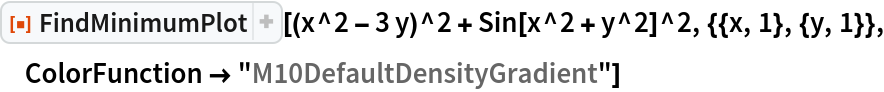Out=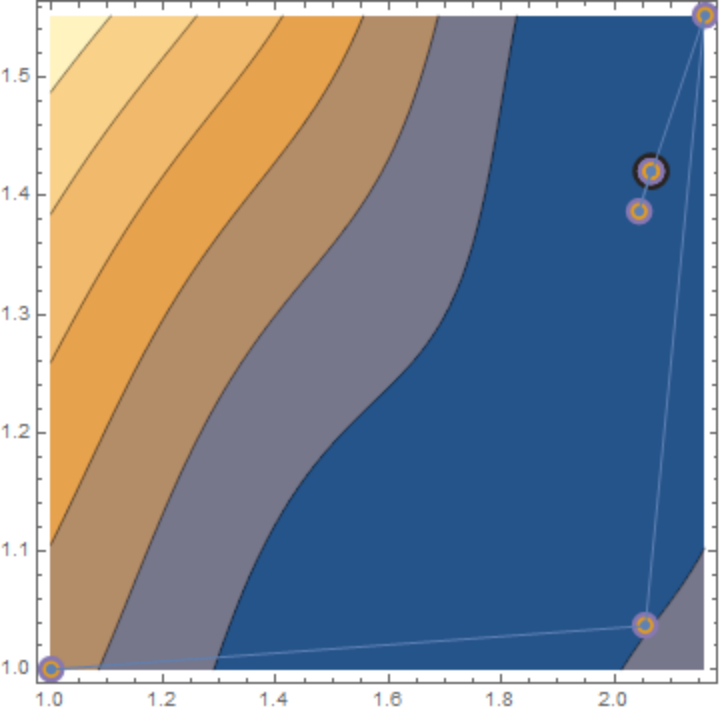#### EvaluationStyles (2)

Use "EvaluationStyles" to change the style used for the evaluation points:

 In:=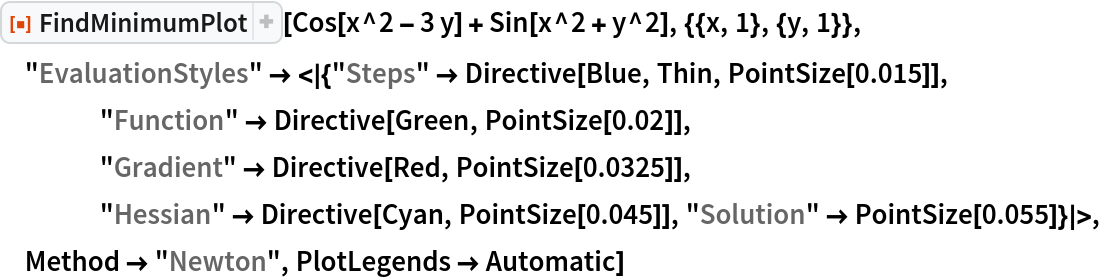Out=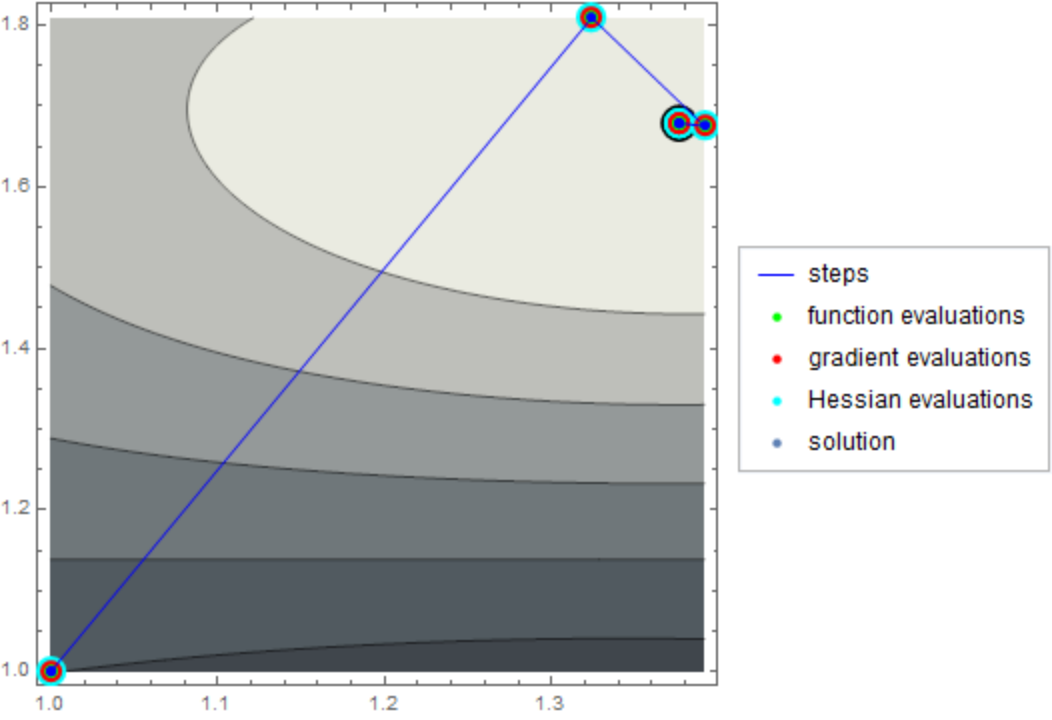Default styles are used if a particular style is left unspecified:

 In:=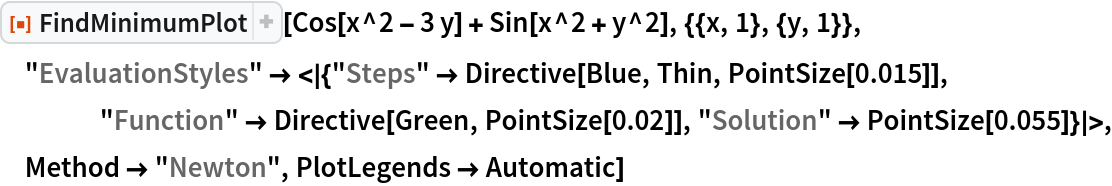Out=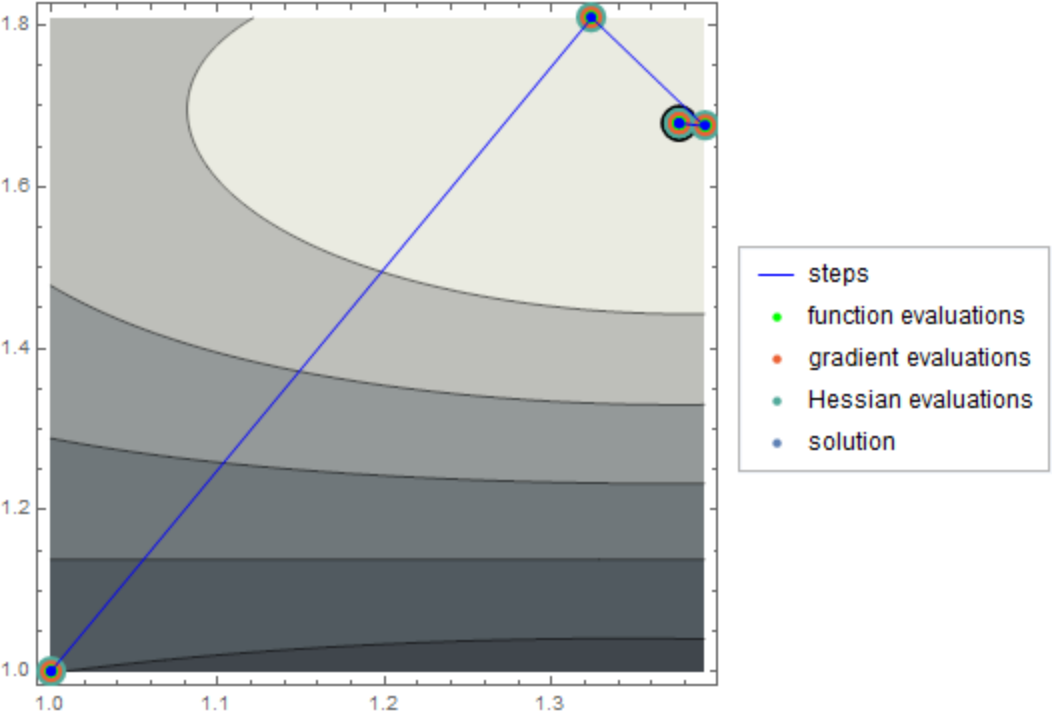#### Method (1)

Compare different minimization methods:

 In:=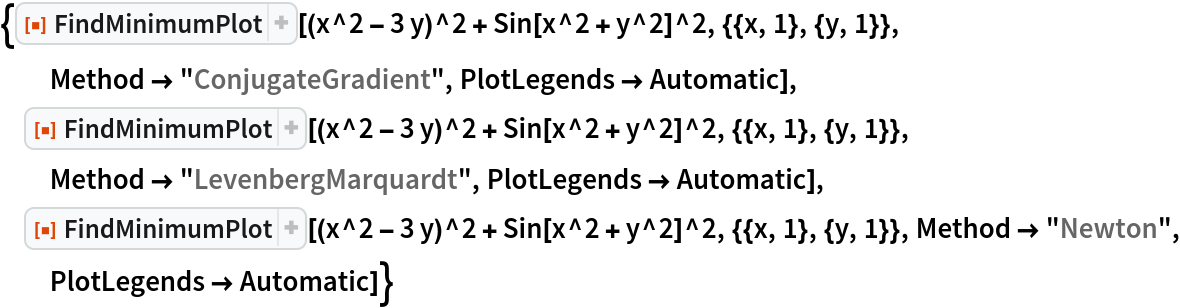Out=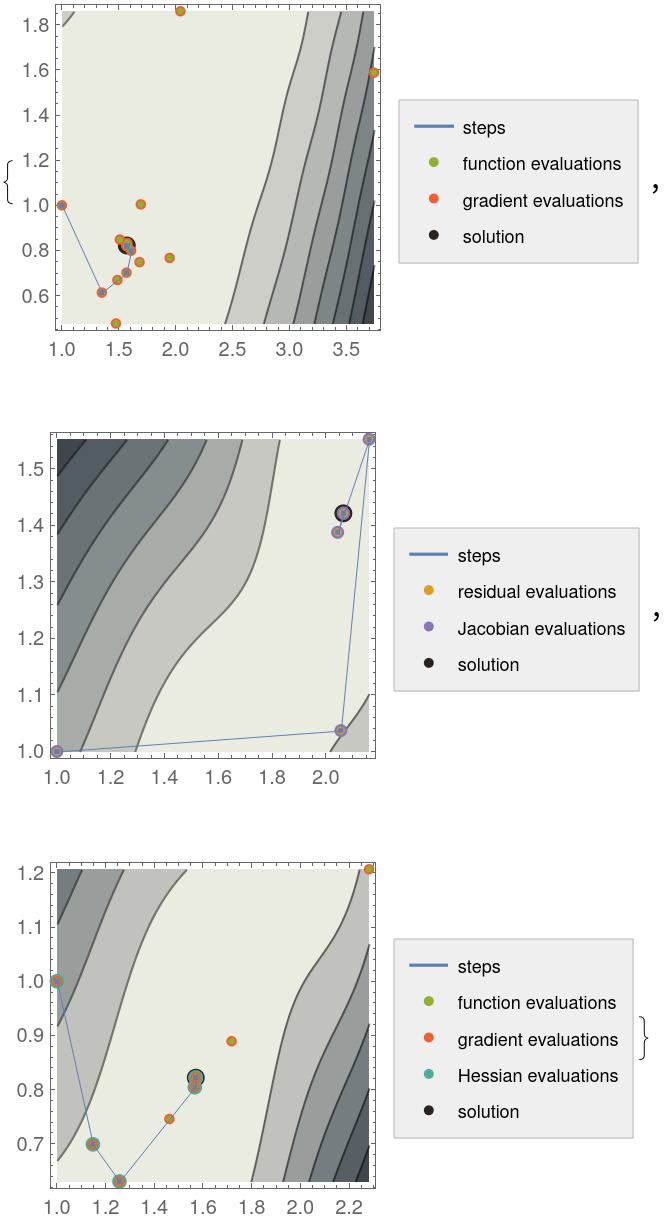#### PlotLegends (1)

Show a legend for the evaluation points:

 In:=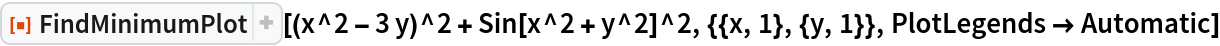Out=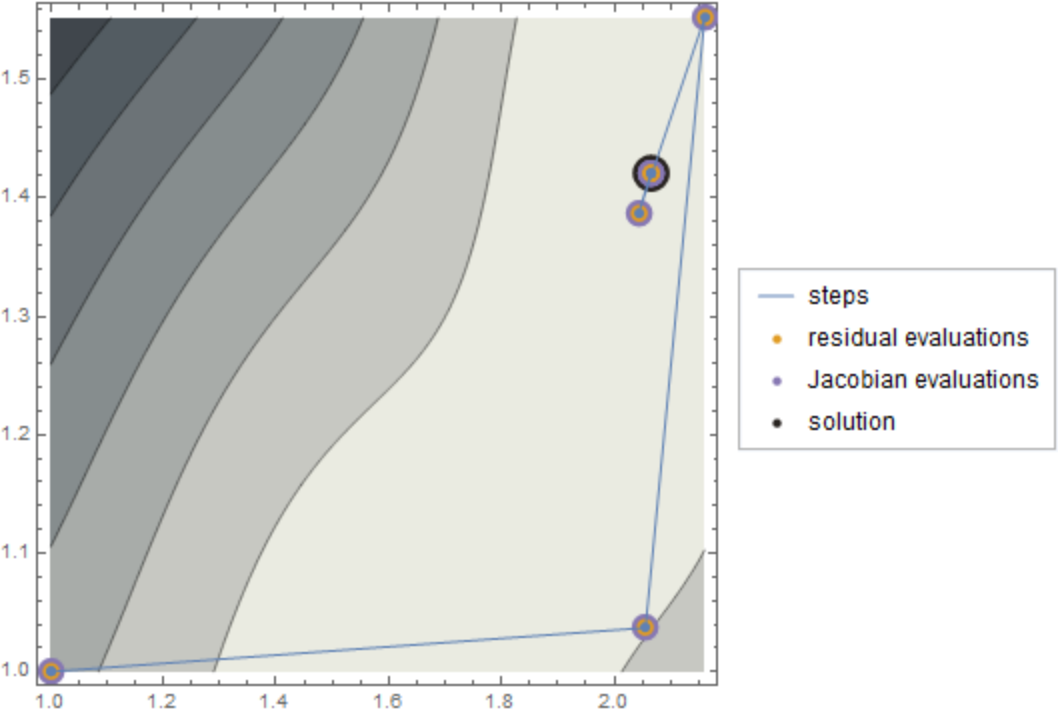## Version History

• 1.0.0 – 16 February 2021